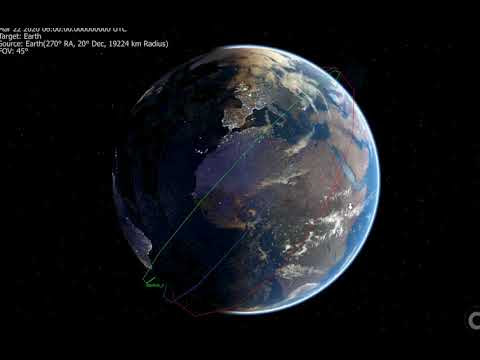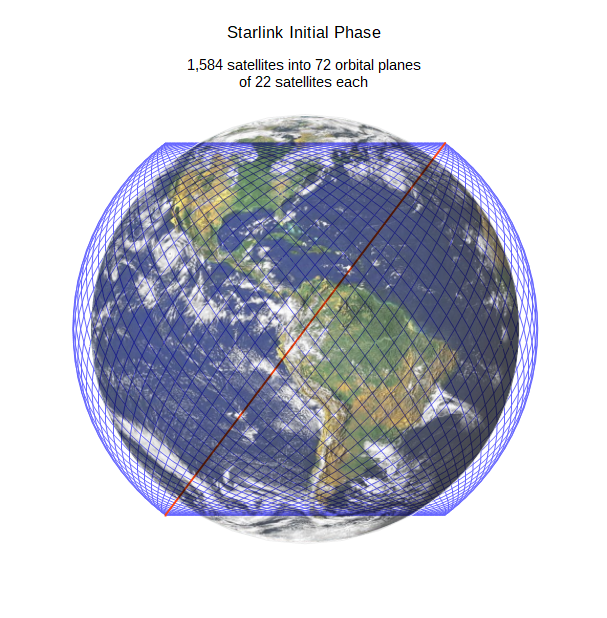## Príspevky

Zobrazujú sa príspevky z dátumu november, 2019

### Nodal precession Precesia uzlovNodal precession is the precession of the orbital plane of a satellite around the rotational axis of an astronomical body such as Earth.Rate of precession The rate of precession depends on the inclination of the orbital plane to the equatorial plane, as well as the orbital eccentricity. For a satellite in a prograde orbit around Earth, the precession is westward (nodal regression), the node and satellite move in opposite directions. A good approximation of the precession rate is {\displaystyle \omega _{\mathrm {p} }=-{\frac {3}{2}}\cdot {\frac {{R_{\mathrm {E} }}^{2}}{\left(a\left(1-e^{2}\right)\right)^{2}}}J_{2}\omega \cos i} where ωp is the precession rate (in rad/s)RE is the body's equatorial radius (6378137 m for Earth)a is the semi-major axis of the satellite's orbite is the